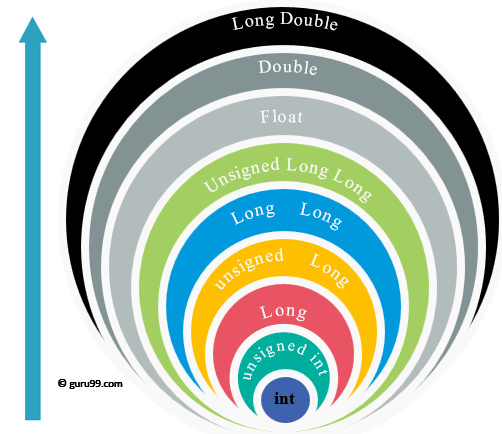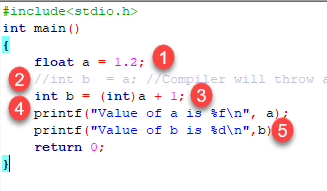# TypeCasting in C: Implicit, Explicit with Example

## What is Typecasting in C?

Typecasting is converting one data type into another one. It is also called as data conversion or type conversion. It is one of the important concepts introduced in ‘C’ programming.

‘C’ programming provides two types of type casting operations:

## Implicit type casting

Implicit type casting means conversion of data types without losing its original meaning. This type of typecasting is essential when you want to change data types without changing the significance of the values stored inside the variable.

Implicit type conversion happens automatically when a value is copied to its compatible data type. During conversion, strict rules for type conversion are applied. If the operands are of two different data types, then an operand having lower data type is automatically converted into a higher data type. This type of type conversion can be seen in the following example.

```#include<stdio.h>
int main(){
short a=10; //initializing variable of short data type
int b; //declaring int variable
b=a; //implicit type casting
printf("%d\n",a);
printf("%d\n",b);
} ```

Output

```10
10
```1. In the given example, we have declared a variable of short data type with value initialized as 10.
2. On the second line, we have declared a variable of an int data type.
3. On the third line, we have assigned the value of variable s to the variable a. On third line implicit type conversion is performed as the value from variable s which is of short data type is copied into the variable a which is of an int data type.

### Converting Character to Int

Consider the example of adding a character decoded in ASCII with an integer:

```#include <stdio.h>
main() {
int  number = 1;
char character = 'k'; /*ASCII value is 107 */
int sum;
sum = number + character;
printf("Value of sum : %d\n", sum );
} ```

Output:

` Value of sum : 108`

Here, compiler has done an integer promotion by converting the value of ‘k’ to ASCII before performing the actual addition operation.

### Arithmetic Conversion Hierarchy

The compiler first proceeds with promoting a character to an integer. If the operands still have different data types, then they are converted to the highest data type that appears in the following hierarchy chart:Consider the following example to understand the concept:

```#include <stdio.h>
main() {
int  num = 13;
char c = 'k'; /* ASCII value is 107 */
float sum;
sum = num + c;
printf("sum = %f\n", sum );} ```

Output:

` sum = 120.000000 `

First of all, the c variable gets converted to integer, but the compiler converts num and c into “float” and adds them to produce a ‘float’ result.

### Important Points about Implicit Conversions

• Implicit type of type conversion is also called as standard type conversion. We do not require any keyword or special statements in implicit type casting.
• Converting from smaller data type into larger data type is also called as type promotion. In the above example, we can also say that the value of s is promoted to type integer.
• The implicit type conversion always happens with the compatible data types.

We cannot perform implicit type casting on the data types which are not compatible with each other such as:

1. Converting float to an int will truncate the fraction part hence losing the meaning of the value.
2. Converting double to float will round up the digits.
3. Converting long int to int will cause dropping of excess high order bits.

In all the above cases, when we convert the data types, the value will lose its meaning. Generally, the loss of meaning of the value is warned by the compiler.

‘C’ programming provides another way of typecasting which is explicit type casting.

## Explicit type casting

In implicit type conversion, the data type is converted automatically. There are some scenarios in which we may have to force type conversion. Suppose we have a variable div that stores the division of two operands which are declared as an int data type.

``` int result, var1=10, var2=3;
result=var1/var2;
```

In this case, after the division performed on variables var1 and var2 the result stored in the variable “result” will be in an integer format. Whenever this happens, the value stored in the variable “result” loses its meaning because it does not consider the fraction part which is normally obtained in the division of two numbers.

To force the type conversion in such situations, we use explicit type casting.

It requires a type casting operator. The general syntax for type casting operations is as follows:

```(type-name) expression
```

Here,

• The type name is the standard ‘C’ language data type.
• An expression can be a constant, a variable or an actual expression.

Let us write a program to demonstrate implementation of explicit type-casting in ‘C’.

```#include<stdio.h>
int main()
{
float a = 1.2;
//int b  = a; //Compiler will throw an error for this
int b = (int)a + 1;
printf("Value of a is %f\n", a);
printf("Value of b is %d\n",b);
return 0;
}```

Output:

```Value of a is 1.200000
Value of b is 2
```1. We have initialized a variable ‘a’ of type float.
2. Next, we have another variable ‘b’ of integer data type. Since the variable ‘a’ and ‘b’ are of different data types, ‘C’ won’t allow the use of such expression and it will raise an error. In some versions of ‘C,’ the expression will be evaluated but the result will not be desired.
3. To avoid such situations, we have typecast the variable ‘a’ of type float. By using explicit type casting methods, we have successfully converted float into data type integer.
4. We have printed value of ‘a’ which is still a float
5. After typecasting, the result will always be an integer ‘b.’

In this way, we can implement explicit type casting in ‘C’ programming.

### Summary

• Typecasting is also called as type conversion
• It means converting one data type into another.
• Converting smaller data type into a larger one is also called as type promotion.
• ‘C’ provides an implicit and explicit way of type conversion.
• Implicit type conversion operates automatically when the compatible data type is found.
• Explicit type conversion requires a type casting operator.

Keep in mind the following rules for programming practice when dealing with different data type to prevent from data loss :

• Integers types should be converted to float.
• Float types should be converted to double.
• Character types should be converted to integer.

#### Top Courses